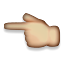## Welcome to the Treehouse Community

The Treehouse Community is a meeting place for developers, designers, and programmers of all backgrounds and skill levels to get support. Collaborate here on code errors or bugs that you need feedback on, or asking for an extra set of eyes on your latest project. Join thousands of Treehouse students and alumni in the community today. (Note: Only Treehouse students can comment or ask questions, but non-students are welcome to browse our conversations.)

### Looking to learn something new?

Treehouse offers a seven day free trial for new students. Get access to thousands of hours of content and a supportive community. Start your free trial today.

# should i multiply or not?

how to do the last one## The questions may not always be asked in the same order.

But most of them involve decoding binary, so I'll give you some hints about that:

Here's one way to decode binary numbers. Make a column for each digit, and starting from the right, put a "1" and then as you move left put double the value of the column next to it. Remember, right-to-left (THIS WAY). You'll get something like this, which is a horizontal version of the helper table shown in the quiz:

```128  64  32  16   8   4   2   1   <-- 8 columns for 8 digits
```

Then, put your binary number on the next line, spread into the columns. Now below that, multiply each top number by the binary digit and put the result below. It's easy, since binary digits are either 1 or 0, so you either put the number on top again or zero.

Then finally, add up all the numbers on the bottom row and that's your answer. Like this:

```128  64  32  16   8   4   2   1   <-- starting columns
0   0   0   0   1   0   0   0   <-- multiply by your binary digits
--- --- --- --- --- --- --- ---
0   0   0   0   8   0   0   0   <-- add these up:  8 + 0 + 0 + 0  =  8
```

Also, since zeros in front don't count you can skip them. So in this case we really only needed 4 columns.